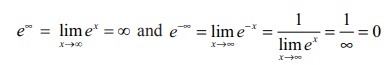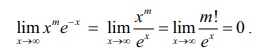Home | | Maths 12th Std | Gamma Integral

# Gamma Integral

Applications of Integration | Mathematics

Gamma Integral

In this section, we study about a special improper integral of the form Ōł×Ōł½0 e ŌłÆx xnŌłÆ1dx , where n is a positive integer. Here, we haveBy LŌĆÖHo╦åpitalŌĆÖsŌĆē rule, for every positive integer m , we get,### Example 9.43

Prove that Ōł×Ōł½0 eŌłÆx xn dx = n!, where n is a positive integer.

### Solution

Applying integration by parts, we getLet In = Ōł×Ōł½0 eŌłÆx xn dx .Then, In = nInŌłÆ1 .

So, we get In = n ( n ŌłÆ1)InŌłÆ2 .

Proceeding in this way, we get ultimately,

In = n ( n ŌłÆ 1)( n ŌłÆ 2) ( 2)(1)I0 .

But, I0 = Ōł×Ōł½0 e ŌłÆx x0dx = ( ŌłÆeŌłÆx)Ōł×0 = 0 +1 = 1 . So, we get In = n (n ŌłÆ 1)(n ŌłÆ 2) (2)(1) = n!.

Hence, we get

Result

Ōł×Ōł½0 e ŌłÆx xn dx = n!, where n is a nonnegative integer.

Note

The integral Ōł×Ōł½0 eŌłÆx xnŌłÆ1dx defines a unique positive integer for every positive integer n Ōēź 1.

Definition 9.1

Ōł×Ōł½0 e ŌłÆx xnŌłÆ1dx is called the gamma integral. It is denoted by ╬ō(n) and is read as ŌĆ£gamma of n ŌĆØ.

NoteExample 9.44

Evaluate Ōł×Ōł½0 e ŌłÆax xn dx ,where a > 0 .

Solution

Making the substitution t = ax , we get dt = adx and x = 0 ŌćÆ t = 0 and x = Ōł× ŌćÆ t = Ōł× .

Hence, we getTags : Applications of Integration | Mathematics , 12th Maths : UNIT 9 : Applications of Integration
Study Material, Lecturing Notes, Assignment, Reference, Wiki description explanation, brief detail
12th Maths : UNIT 9 : Applications of Integration : Gamma Integral | Applications of Integration | Mathematics